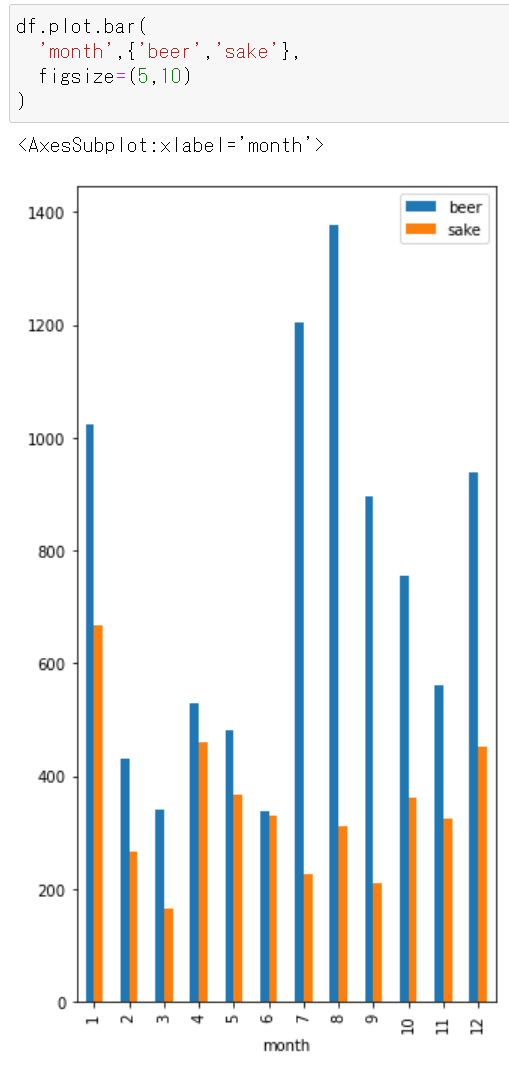Thing you want to do

• I want to read CSV data with pandas and display a bar graph.
• I want to customize the color and size of the graph.
• Since the purpose is to learn basic operations, the dataset handles simple contents created by yourself.
• Here, group by etc. is not used because the purpose is not "aggregation" but "visualization" of data.

Premise

• The jupyter environment has already been built.
• You have installed the required python library.

Official documentation

Official pandas documentation. Specifications of plot.bar.

https://pandas.pydata.org/pandas-docs/stable/reference/api/pandas.DataFrame.plot.bar.html

CSV dataset

sample.csv

Fictitious data created by myself. Shows the sales of companies that sell alcoholic beverages. Monthly sales amount of beer and sake (100 million yen). For example, in February, beer (beer) sold 43.1 billion yen and sake (sake) sold 26.7 billion yen.

month,beer,sake
1,1024,667
2,431,267
3,341,166
4,530,461
5,482,367
6,339,331
7,1203,227
8,1376,312
9,896,211
10,754,361
11,561,325
12,938,452

Below, implementation in python.

Import the library

import pandas as pd
import matplotlib as mpl
import matplotlib.pyplot as plt

The contents of df are as follows.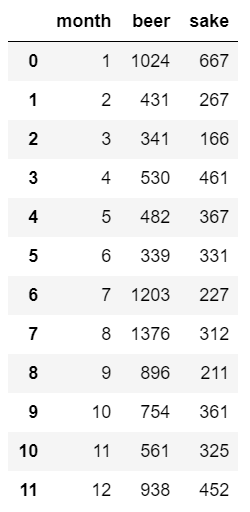View monthly beer sales

df.plot.bar('month', 'beer')

The horizontal axis is "month" and the vertical axis is "beer sales amount (100 million yen)".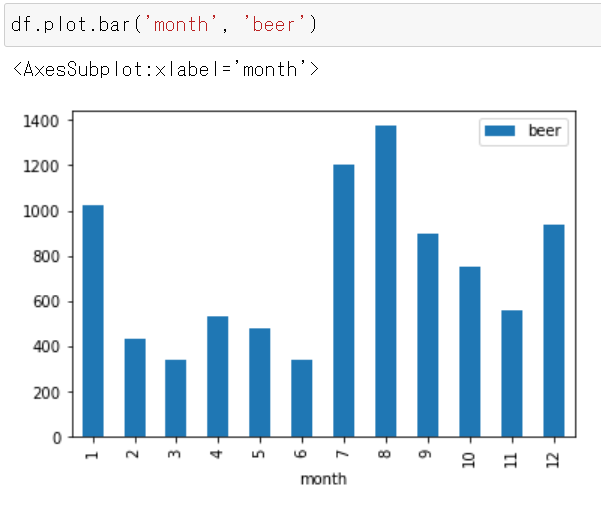• It can be seen that August sales were the highest (137.6 billion yen).

View monthly sake sales

df.plot.bar('month', 'sake')

The horizontal axis is "month" and the vertical axis is "sake sales amount (100 million yen)".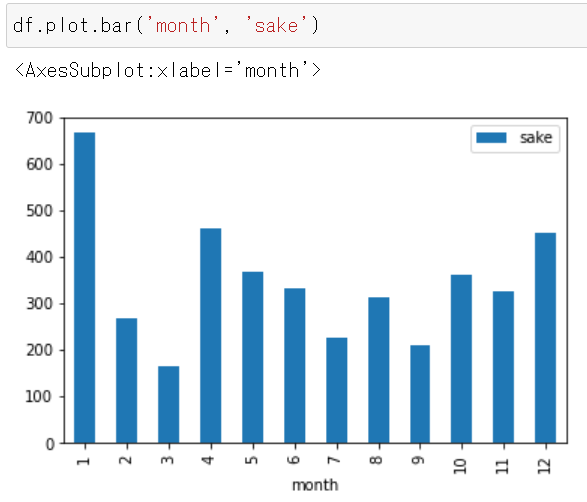• It can be seen that January sales are the highest (66.7 billion yen).

Display monthly "Beer and Sake Sales"

df.plot.bar('month',{'beer','sake'} )

The horizontal axis is "month" and the vertical axis is "sales amount of beer (blue) and sake (orange) (100 million yen)".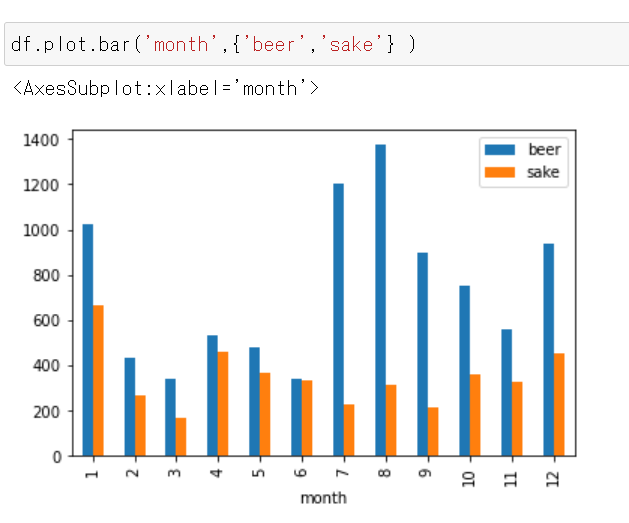You can see the following.

• Beer sales are highest in August, but sake sales are highest in January.
• Beer sells more than sake.

Display monthly "sales of beer and sake" (stacked)

Add stacked = True to make a stacked graph.

df.plot.bar('month',{'beer','sake'}, stacked=True)

The horizontal axis is "month" and the vertical axis is "sales amount (100 million yen) of beer (blue) and sake (orange)" (accumulated).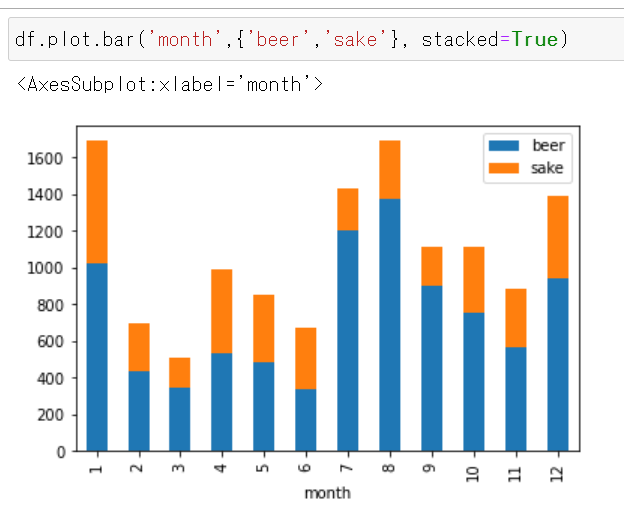You can see the following.

• In total, beer and sake sell best in January and August.
• Beer has a higher ratio to total sales than sake.

Change the color of the stick

• If you want beer to be red and sake to be green.
df.plot.bar(
'month',{'beer','sake'},
color={"beer": "red", "sake": "green"}
)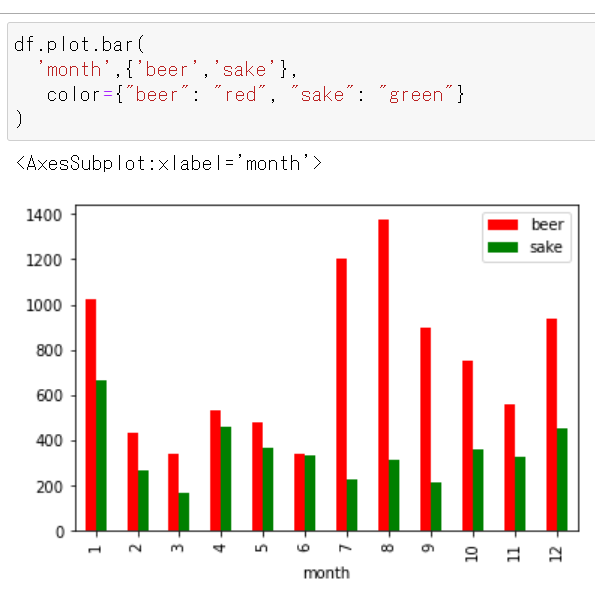Stack

df.plot.bar(
'month',{'beer','sake'},
color={"beer": "red", "sake": "green"},
stacked=True
)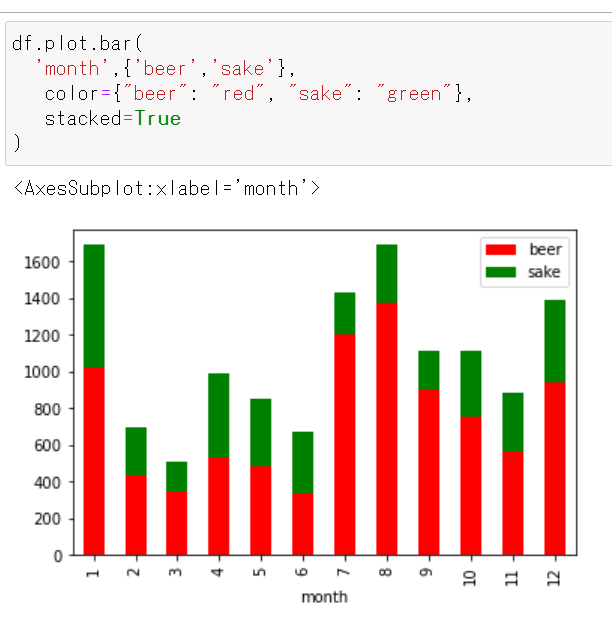Scale the size of the graph display area

Specify with figsize.

figsize=(5,5)

df.plot.bar(
'month',{'beer','sake'},
figsize=(5,5)
)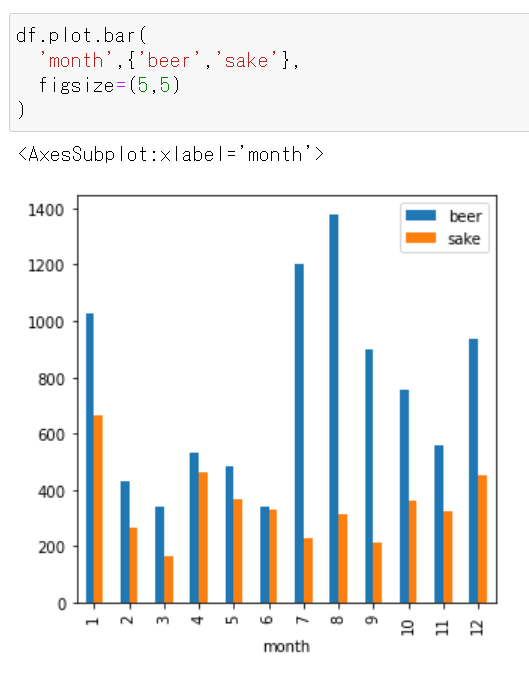figsize=(8,5)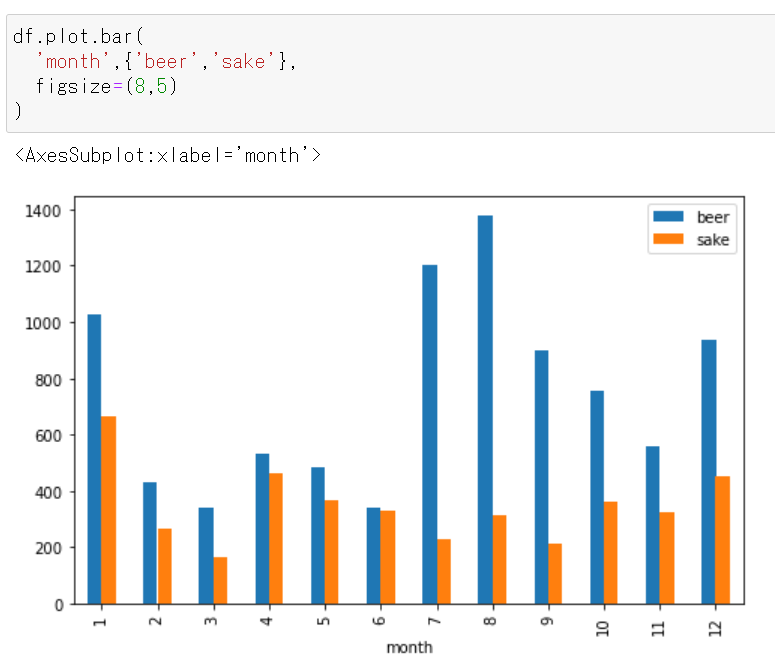figsize=(5,10)